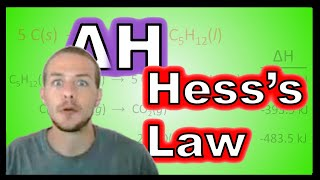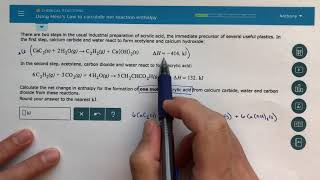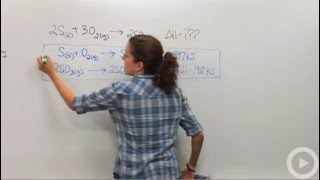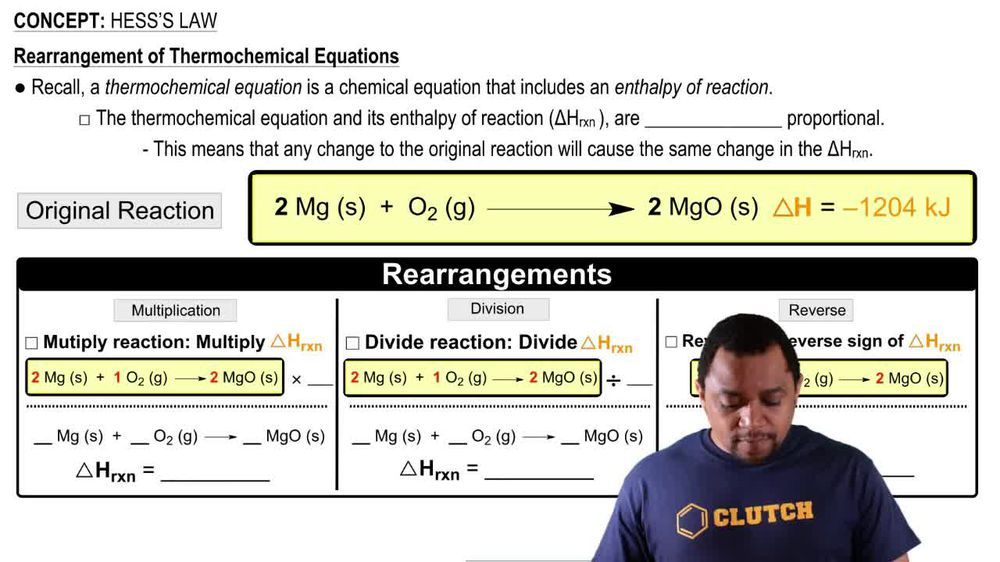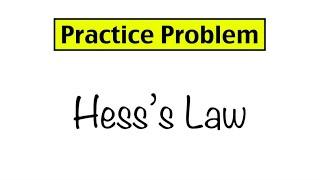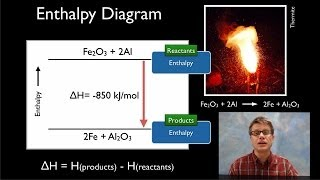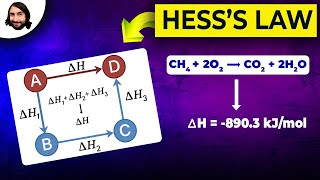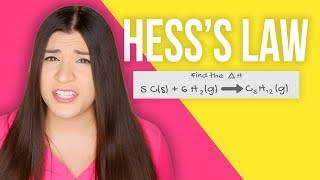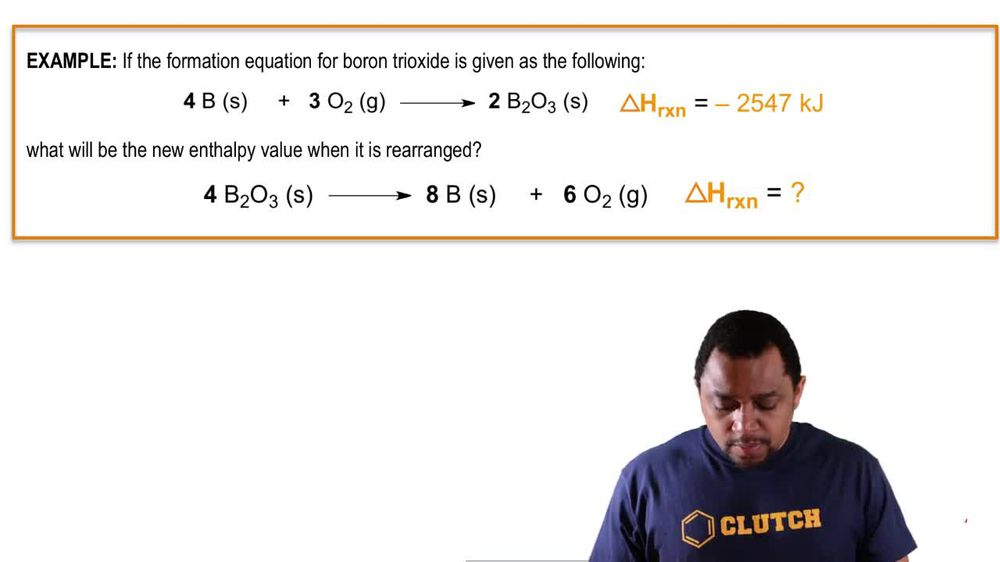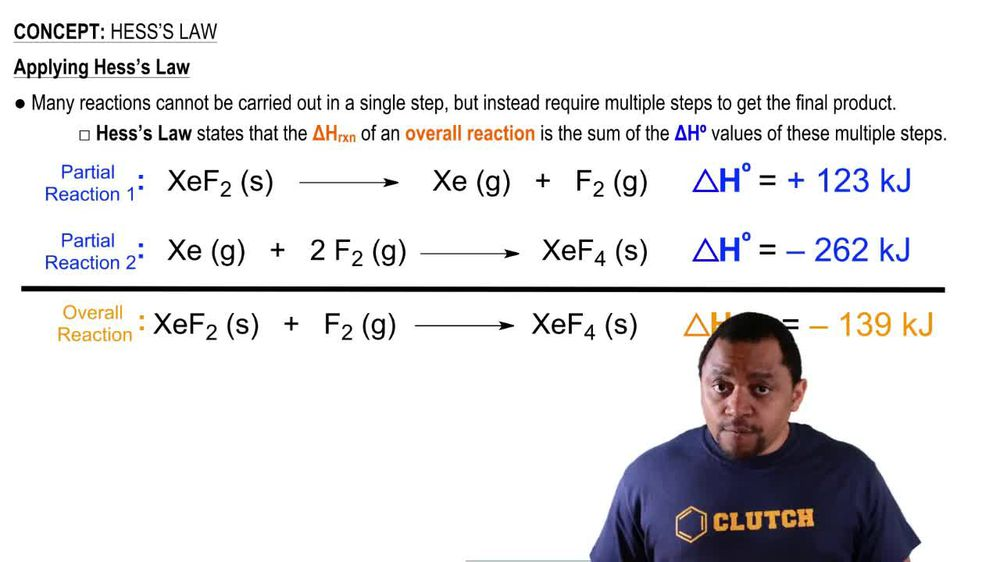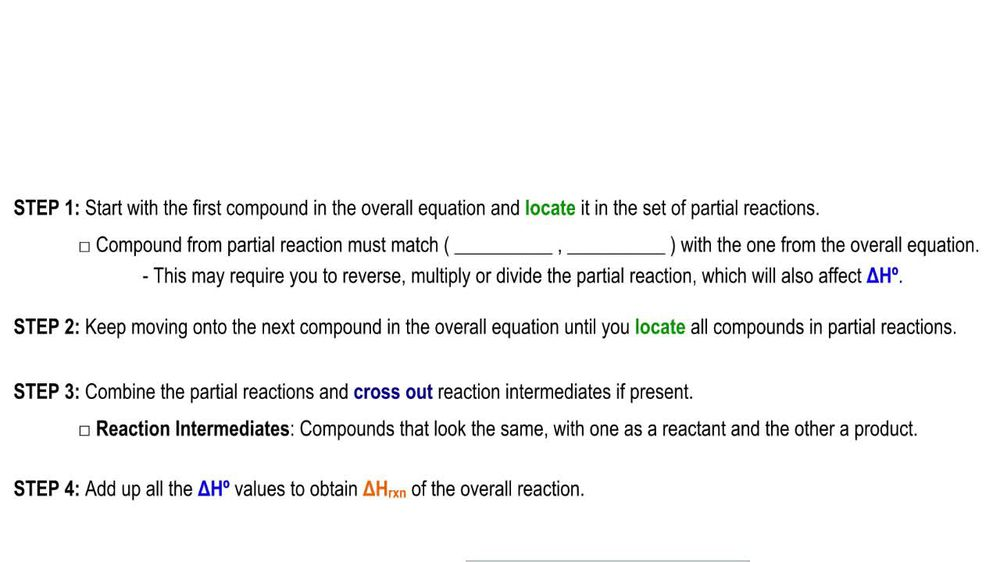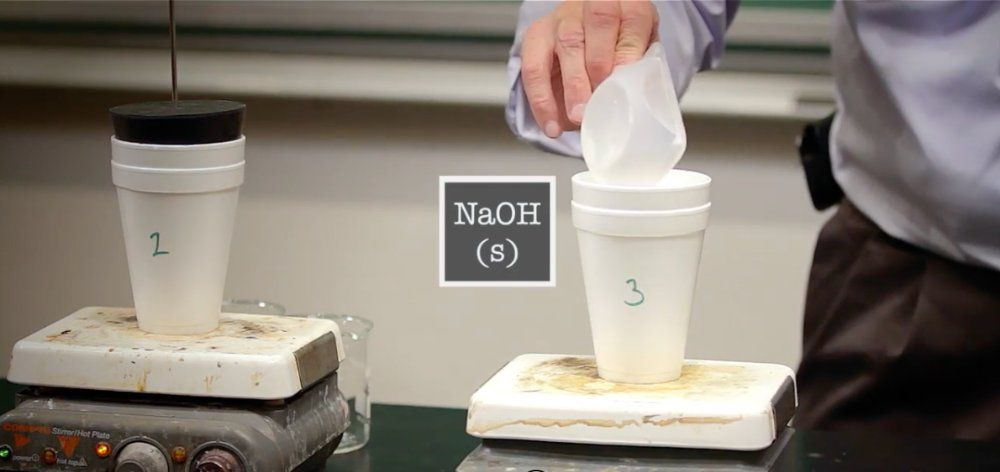Start typing, then use the up and down arrows to select an option from the list.
1. 8. Thermochemistry2. Hess's Law# Hess's Law

by Jules Bruno
267 views
2
1
2
Now, Hess's law has to do with the rearrangement of thermo chemical equations to help us find the overall entropy of reaction. Now recall. A thermal chemical equation is a chemical equation that involves or includes an entropy of reaction. Now we're going to say the thermal chemical equation, and it's NLP of reaction, which is Delta h r x n are directly proportional. Okay, this means that any change to the original equation will cause the same change within your entropy of reaction. So let's say we have an original equation here. Original thermal chemical equation where we have two moles of magnesium, a solid plus o two gas, and it produces two moles of magnesium oxide. The entropy associated with this is negative. 12 04 killer jewels. Now I can do different things different rearrangements to this thermal chemical equation that will affect it but also affect my delta h of reaction. So the things I can do is I can multiply this equation by a value I can divide it by a number, or I can reverse the reaction. So let's say we multiply it here we have our original equation and let's say multiply it by two. Now, if I multiply it by two, the coefficients not become 42 and four. If I multiply the equation by two, then that means I also have to multiply my delta H by two. So this original value of negative 12 04 killer Jules gets multiplied by two. Okay? And now my new entropy of the reaction is negative. 24 08 killer jewels. Now, let's say instead of multiplying, I divided. So my original equation again. Now I, divided by two, dividing it by two changes my coefficients now to one toe, one for magnesium, solid to one half for and one for magnesium oxide. Again, Whatever I do to the equation, I must do the same thing to my delta h So negative 12 04 gets divided by two. So it becomes negative. 602 killer jewels. Is it? Finally, the last thing I could do to my thermo chemical equation? As I can reverse it, what was a product can become a reactant. So that's two m g o solid. And what was a reactant cannot become products. So two mg solid + Now, when I reverse the reaction. What that does is it reverses the sign of Delta H. So it was a negative 12. 04 Now it's gonna become a positive 12. 04 So just realize that if I reverse the reaction, I reverse the sign of Delta H. So just remember, these are the three things I could do toe a thermal chemical equation, and they have a direct impact on my delta h of reaction for that particular thermal chemical equation.